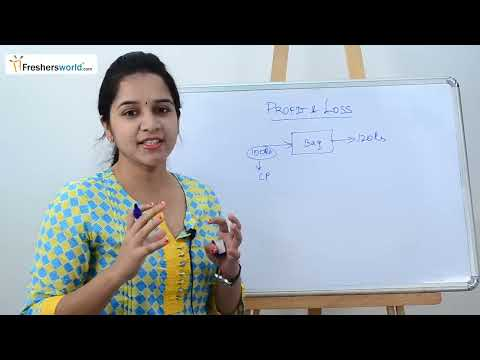# Profit and Loss

An income statement or profit and loss account (also referred to as a profit and loss statement (P&L), statement of profit or lossrevenue statementstatement of financial performanceearnings statementstatement of earningsoperating statement, or statement of operations) is one of the financial statements of a company and shows the company’s revenues and expenses during a particular period.

##Cost Price ( CP): Price at which the seller brought the product. Selling Price ( SP): Price at which the seller sells the product to you or the customer. Marked Price ( MP): It is the price displayed on the product. ( Also, called MRP ) Profit ( P): When SP > CP, the seller makes a profit. P = SP – CP ; P% = ( P / CP ) x 100

• Loss ( L ) : When SP < CP, seller suffers a loss.

L = CP – SP ; L% = ( P / CP ) x 100 Remember that both profit and loss are always calculated on the cost price of the product. Discounts ( D): The concession given by the seller on the market price ( MP ) of the product is called discount. SP = MP – Discount Markup = MP – CP ; Markup % =  [ ( MP – CP ) / CP ] x 100

• Remember that discount percentages are always calculated on the Marked Price ( MP ) of the product.

The main formula for this is as follows : Initial Value ( CP ) x Multiplication Factor ( MF ) = Final Value ( SP ) For two successive profits or losses on an item, the formula for the resultant profit/loss % is:-   x + y + ( xy / 100) Note: Both ‘x’ and ‘y’, here are profit/loss percentages, as given in the question. For-profit use ‘+’ sign and for loss use ‘–’ sign. If the result is in + then, there is an overall profit, if it is in ‘–’ then there is an overall loss. For more than two successive profits or losses on an item, the above formula for resultant profit/loss % is applied iterative-ly.

• If two items, which have the same CP, are sold such that one is at a profit of x% whereas the other is at a loss of x%. Then in the entire transaction, the seller neither suffers a loss nor receives profit.
• However, if two items with the same SP, are sold such that one is at a profit of x% whereas the other is at a loss of x%. Then in the entire transaction, the seller suffers a loss, which is given by the following formula:-

Overall Loss% = x^2 / 100  [ square of the common profit or loss % divided by 100 ]